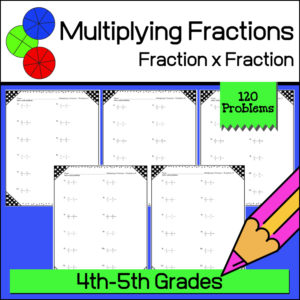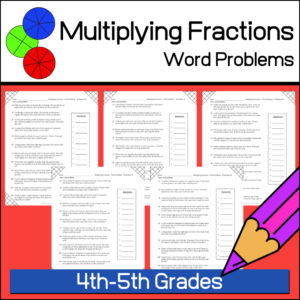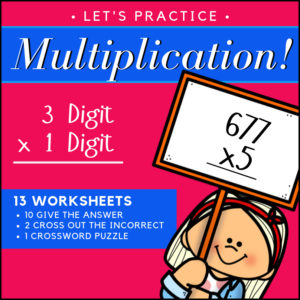Showing 1–20 of 35 results

•\$3.00

This resource is designed to give students the practice the need to become proficient at multiplying decimals. There are three levels, 15 worksheets (16 problems each) and answer keys. That’s 240 problems – EACH WORKSHEET includes a different growth mindset quote!
(See description below for more details)

•\$3.00

This resource is designed to give students the practice the need to become proficient at multiplying decimal numbers. There are three levels, 15 worksheets (20 problems each) and answer keys. That’s 300 problems! Use as practice sheets, homework, or for quizzes and tests.

Also included: Visual poster to help students with ‘where to place’ the decimal point in their answers.

3 Levels:

• Level 1 (5 worksheets)
Tenths multiplied by tenths
• Level 2 (5 worksheets)
Tenths multiplied by hundreds and/or by thousandths
Hundredths multiplied by hundredths and/or by thousandths
• Level 3 (5 worksheets)
Mixed from tenths/hundredths/thousandths multiplied by tenths/hundredths/thousandths
•\$3.00

Give your students the practice and reinforcement they need as they are learning to multiply fractions with fractions! This resource includes 120 problems (10 worksheets with 12 problems each) and answer keys.

•\$3.00

Help students develop their math word problem skills and practice solving multiplying fractions all at the same time! This resources provides ten (10) ready to use, print and go worksheets each with 12 word problems for a total of 120 problems (answer keys provided).

•\$2.50

Give your students the practice and reinforcement they need as they are learning to multiply fractions by whole numbers! This resource includes 100 problems (5 worksheets with 20 problems each) and answer keys.

•\$3.00

Help your students understand and remember the basis of multiplying fractions using a visual model method! This product, Multiplying Fractions with Visual Models #2, gives you 110 problems in which students will multiply a fraction with a another fraction. For each problem students will be asked to color in given visual fraction models to find the answer before giving the answer.

Product includes 10 worksheets (11 questions each) = 110 questions + answer keys.

• Sale!\$9.00 \$7.00

I’ve bundled 3 of my products for students working with decimals! Resource #1 will help students understand equivalent fractions, decimals and percents. Resource #2 will have students working with decimal notation, location on a number line, digit value and relative value in relation to half. Resource #3 will give students a lot of practice adding and subtracting decimals and includes 100 word problems!

There are over 700 problems included in this bundle and a lot of work with visual models. Answer Keys are provided.

•\$2.00

This resource will help students understand equivalent fractions, decimals and percents. It includes a poster / handout of explanation and a one page student worksheet. When given a fraction, decimal or percent, students will be tasked with providing the equivalent fraction, decimal or percent. Answer Key provided.

•\$2.50

4 Christmas themed Math worksheets for students to practice changing fractions into decimals.

• Worksheet #1
Fractions with denominators of 2, 4, 5 and 10
• Worksheet #2
Fractions with denominators of 3, 5, 6, 7, 9 and 10
• Worksheet #3
Fractions with denominators of 10, 100 and 1000
(Highest fraction 694/1000)
• Worksheet #4
Fractions with denominators of 10, 100 and 1000
(Will include mixed fractions – example: 3337/1000)

•\$3.00

15 Math worksheets for students to practice and show their understanding of equivalent fractions! Answer Keys provided

Resource includes:

• 10 worksheets requiring students to reduce a fraction to the lowest equivalent fraction
• 3 worksheets asking students to choose the equivalent fraction from a given set
• 2 worksheets where students are to complete a pattern by writing in the missing equivalent fraction.
•\$3.00

15 Christmas themed Math worksheets for students to practice and show their understanding of equivalent fractions! Answer Keys provided

Resource includes:

• 10 worksheets requiring students to reduce a fraction to the lowest equivalent fraction
• 3 worksheets asking students to choose the equivalent fraction from a given set
• 2 worksheets where students are to complete a pattern by writing in the missing equivalent fraction.
•\$2.25
•\$3.25

This resource includes 27 ready to use Math worksheets for 4th-5th grade students. Designed to give students plenty of practice and to reinforce student knowledge of decimal notation, location on a number line, digit value and relative value in relation to half.

•\$3.00

Help your students understand and remember the basis of multiplying fractions using a visual model method! This product gives you 120 problems in which students will multiply a fraction with a whole number. For each problem students will be asked to color in given visual fraction models to find the answer. Then they are to write the answers using mixed numbers.

Product includes 10 worksheets (12 questions each) = 120 questions + answer keys.

•\$2.00

4 Fraction Wall posters (or handouts)! You’ll get 2 in color (one with fractions and one without) and 2 in b/w (also one with fractions and one without.

•\$3.00

Give your students the practice they need in identifying factors with this 4th grade Math resource! Students with be asked to find all factor pairs for a whole number within the range of 1-100. I’ve created 10 different worksheets (130 problems in all). As an added bonus, I’ve included two copies of each: One without and one with a cute graphic and a positive, growth mindset quote!

⭐ Why have I added mindset quotes to a Math resource? Research shows that it’s the way students think about their ability that really counts. So as students practice and reinforce their Math skills, their thoughts about their own abilities and possibilities can be increased through the reinforcement of the mindset quotes!

•\$4.99

Give your students a fun way to practice their multiplication facts! Your students will love this fast paced math game with included fun twists such as: the ‘Green Horn‘, ‘Stampede!’, ‘Playing in the Mud‘, ‘Stomped‘ and ‘Growing Herd‘. Your students are going to want to play this game again and again!

Includes:

• – Game instructions
• – Game Cards (all facts 0-12 PLUS fun ‘twist’ game cards)
• – (Optional) Card backs
•\$1.25

Studying Roman Numerals? Display this colorful poster on a bulletin board or in a Math center! Includes roman numerals for 1, 5, 10, 50, 100, 500 and 1,000.

•\$2.25

These 300 problems will give your students the practice they need to reinforce their multiplication skills (single digit number x double digit)! This resource includes 10 worksheets with 30 problems each. Each can be used by students for practice or for assessment (quiz and test). Answer keys provided.

Skill: Students will multiply a two digit whole number by a one-digit whole number.

•\$4.99

OVER 1,800 problems, your students will have the practice they need to understand and learn their multiplication facts 0 to 10!

The resource begins with repeated addition (to reinforce the concept of addition in relation to multiplication) and ends with students completing all multiplication facts.